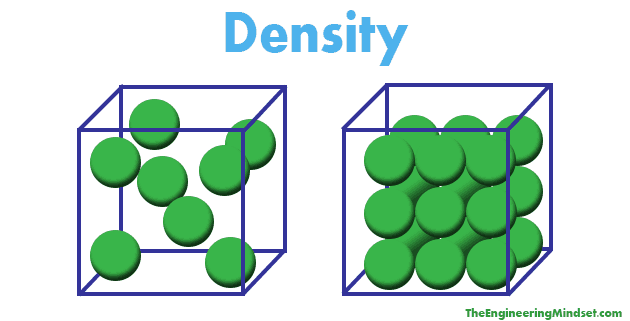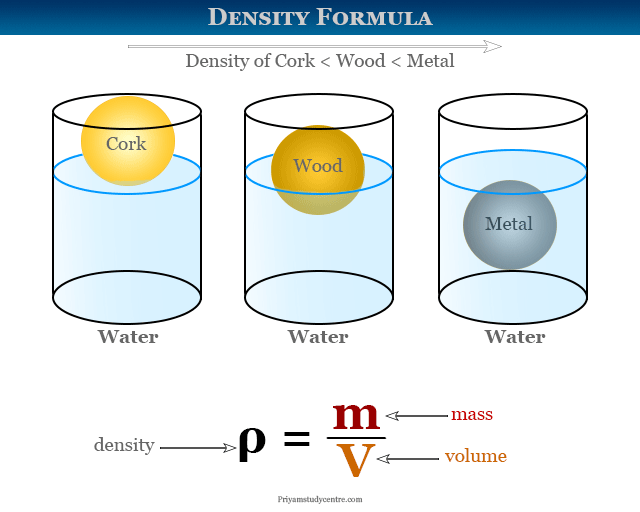Gayathri Mani

HS-PS1-3

# Density Study Guide

Density is defined as the mass per unit volume of a substance.

Table of content

## INTRODUCTION

Have you seen a log of wood floating on the surface of the water? Have you ever successfully been able to mix oil and water? Well, everything that we can see in our daily lives is due to the weight and density of the substance. As the density of wood is lower than water, it can freely float on the water. The same goes for the oil, which does not get mixed or dissolved in water. Now the question arises — what is density? So let’s briefly look at the density and some density problems in chemistry.Source

### DENSITY: DEFINITION

Density is defined as mass per unit volume, i.e.; we can find out the density of a substance if we divide the mass by the volume of the substance.

The formula is: D = M / V

It is easier to find the volume of regular shape objects like cubes, cones, triangles, etc., using the formulas. But, for irregularly shaped objects, it is not very easy. Hence, the best way is to use the irregular-shaped object to displace a liquid medium, be it water, mercury, etc., and get the value of the displaced liquid. That will specify the volume of the shape.Source

### STEPS TO THE CALCULATED DENSITY OF AN OBJECT

It is easier to calculate the density of an object if you are aware of the mass and volume. But you need to remember these steps before solving the problem:

Step 1: Read the problem sum thoroughly. See what the questions want you to find out.

Step 2: Check the units of the given data. Check if the question has specified you to an in any other unit.

Step 3: Do the required conversions.

Step 4: Then, put the values and find out the density.

Let us try out some of the density practice problems!

### DENSITY PRACTICE PROBLEMS CHEMISTRY

You have a sample of granite with a density of 2.8 g/cm³. The density of water is 2.0 g/cm³. What is the specific gravity of your granite?

Solution: Specific gravity is the density of the substance divided by the density of water.

Specific Gravity = Density object / density of water

Specific Gravity = 2.8 / 2 = 1.4

The units get canceled out; hence, specific gravity is unitless.

### FAQs:

1. How do you solve density problems in chemistry?

• Firstly, as you know, the formula of density which is mass per unit volume, D = M / V, use this formula in the problems.
• Secondly, You should check the mass and unit of the volume and then solve the problem.

2. You have a rock with a volume of 18 cm3 and a mass of 45 g. What is its density?

Density = Mass / Volume

D = 45 g / 18 cm³ = 2.5 g / cm³

3. A solution of water and salt contains 35 g of salt in 350 mL of water. What is the density of saltwater? (Use density of water = 1 g/mL)

Given:

Mass of salt = 35 g

The volume of water = 350 mL

Let us find out the mass of water:

The density of water = Mass of water/volume of water

Mass of water = density of water x volume of water

∴ Mass of water = 1 x 350 = 350 g

Total mass of the solution = mass of salt mass of water

Total mass of the solution = 35 350 = 385 g

Volume of the solution = 350 mL

∴ density of salt water = 385 / 350 = 1.1 g/mL

The density of saltwater is 1.1 g.mL.

We hope you enjoyed studying this lesson and learned something cool about Density! Join our Discord community to get any questions you may have answered and to engage with other students just like you! We promise it makes studying much more fun! 😎

## SOURCES

1. How to Calculate Density?: https://www.thoughtco.com/how-to-calculate-density-609604#:~:text=Key%20Takeaways%3A%20How%20to%20Calculate%20Density&text=The%20density%20equation%20is%20density,proper%20mass%20and%20volume%20units.Accessed 7th March 2022.
2. Density: https://serc.carleton.edu/mathyouneed/density/densitysp.html. Accessed 7th March 2022.